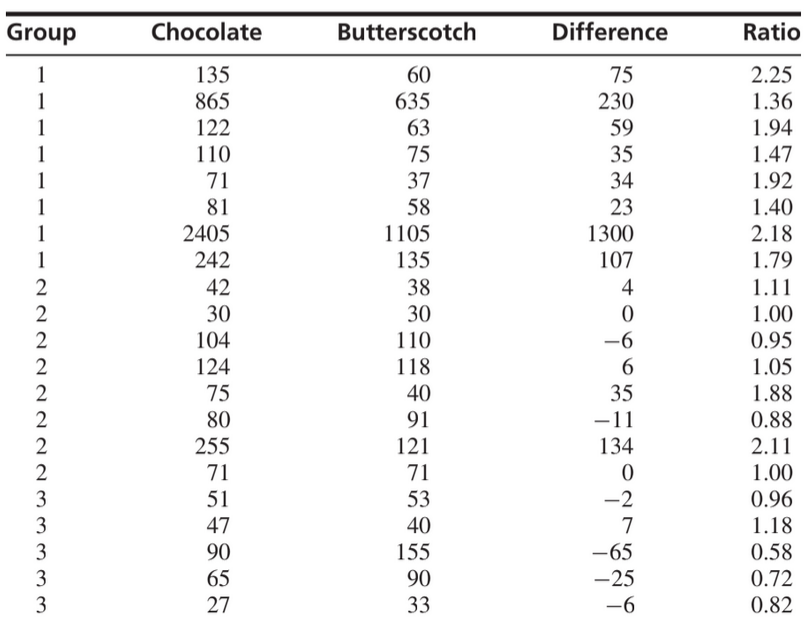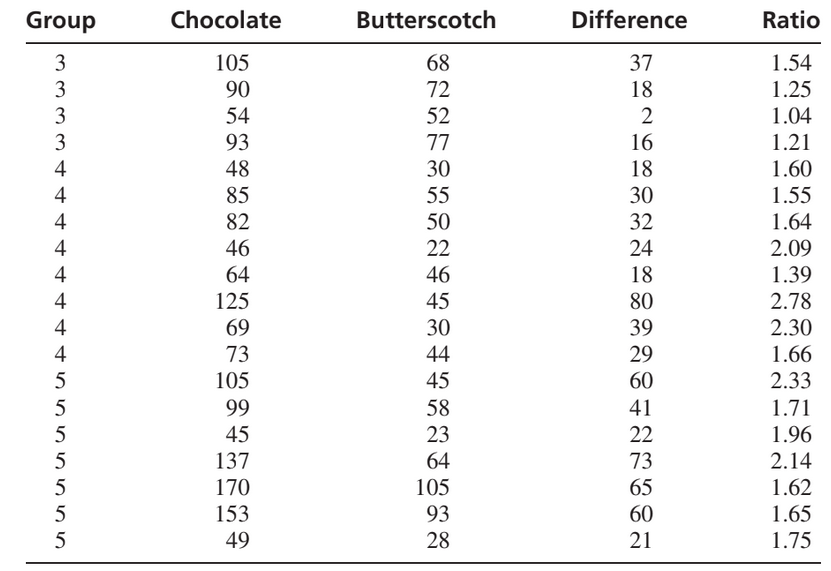×
Get Full Access to Statistics For Engineers And Scientists - 4 Edition - Chapter 9 - Problem 5se
Get Full Access to Statistics For Engineers And Scientists - 4 Edition - Chapter 9 - Problem 5se

×

# An experiment was performed to determine whether differentISBN: 9780073401331 38

## Solution for problem 5SE Chapter 9

Statistics for Engineers and Scientists | 4th Edition

• Textbook Solutions
• 2901 Step-by-step solutions solved by professors and subject experts
• Get 24/7 help from StudySoup virtual teaching assistantsStatistics for Engineers and Scientists | 4th Edition

4 5 1 243 Reviews
29
4
Problem 5SE

An experiment was performed to determine whether different types of chocolate take different amounts of time to dissolve. Forty people were divided into five groups. Each group was assigned a certain type of chocolate. Each person dissolved one piece of chocolate, and the dissolve time (in seconds) was recorded. For comparison, each person in each group also dissolved one piece of butterscotch candy; these pieces were identical for all groups. The data, which include the group, the dissolve times for both chocolate and butterscotch, the difference between the dissolve times, and the ratio of the dissolve times, are presented in the following table. Note that the design is slightly unbalanced; group 3 has nine people and group 5 has only seven.To test whether there are differences in the mean dissolve times for the different types of chocolate, someone suggests performing a one-way ANOVA, using the dissolve times for the chocolate data. Do these data appear to satisfy the assumptions for a one-way ANOVA? Explain. Someone else suggests using the differences (Chocolate − Butterscotch). Do these data appear to satisfy the assumptions for a one-way ANOVA? Explain. Perform a one-way analysis of variance using the ratios. Can you conclude that the mean ratio of dissolve times differs for different types of chocolate?Step-by-Step Solution:
Step 1 of 3

Week 7 Lecture 7 March 8th, 2017 Review: Population and Sample Researchers often want to answer questions about some large groups of individuals. (This group is called the population.) Often the researchers cannot measure (or survey) all individuals in the population, so they measure a subset of individuals that is chosen to represent the entire population. (This subset is called a sample.) A sample design describes exactly how to choose a sample from the population The researchers then use statistical techniques (Called statistical inference) to make conclusions about the population based on the sample Choosing a Representative Sample Sample Survey: Selects a sample from the population of all individuals about which we desire information. How do we decide which individuals to include in the sample Goals: Individuals in sample are representative of the population (Provide accurate information about the population) Minimize cost of obtaining the sample (Money, Time, Personnel, etc.) Biased Sample A sample is biased if it is not representative of the population Biased samples tend to systematically overrepresent certain segments of the population and systematically under-represent other segments Important: Because a biased sample does not represent the entire population, cannot accurately infer anything about the population of interest from such a sample.

Step 2 of 3

Step 3 of 3

##### ISBN: 9780073401331

This full solution covers the following key subjects: chocolate, dissolve, Group, times, butterscotch. This expansive textbook survival guide covers 153 chapters, and 2440 solutions. This textbook survival guide was created for the textbook: Statistics for Engineers and Scientists , edition: 4. The answer to “?An experiment was performed to determine whether different types of chocolate take different amounts of time to dissolve. Forty people were divided into five groups. Each group was assigned a certain type of chocolate. Each person dissolved one piece of chocolate, and the dissolve time (in seconds) was recorded. For comparison, each person in each group also dissolved one piece of butterscotch candy; these pieces were identical for all groups. The data, which include the group, the dissolve times for both chocolate and butterscotch, the difference between the dissolve times, and the ratio of the dissolve times, are presented in the following table. Note that the design is slightly unbalanced; group 3 has nine people and group 5 has only seven. 1. To test whether there are differences in the mean dissolve times for the different types of chocolate, someone suggests performing a one-way ANOVA, using the dissolve times for the chocolate data. Do these data appear to satisfy the assumptions for a one-way ANOVA? Explain. 2. Someone else suggests using the differences (Chocolate ? Butterscotch). Do these data appear to satisfy the assumptions for a one-way ANOVA? Explain. 3. Perform a one-way analysis of variance using the ratios. Can you conclude that the mean ratio of dissolve times differs for different types of chocolate?” is broken down into a number of easy to follow steps, and 215 words. The full step-by-step solution to problem: 5SE from chapter: 9 was answered by , our top Statistics solution expert on 06/28/17, 11:15AM. Statistics for Engineers and Scientists was written by and is associated to the ISBN: 9780073401331. Since the solution to 5SE from 9 chapter was answered, more than 369 students have viewed the full step-by-step answer.

## Discover and learn what students are asking

Calculus: Early Transcendental Functions : Differential Equations: Separation of Variables
?In Exercises 1-14, find the general solution of the differential equation. $$\frac{d y}{d x}=\frac{6-x^{2}}{2 y^{3}}$$

Statistics: Informed Decisions Using Data : Inference about the Difference between Two Medians: Dependent Samples
?Rat Hemoglobin Hemoglobin helps the red blood cells transport oxygen and remove carbon dioxide. Researchers at NASA wanted to discover the effects of

Unlock Textbook Solution